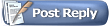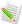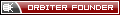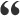Orbiter-ForumYet another propagation problem (atmospheric drag mostly)
 User Name Remember Me? Password
 Register Blogs Orbinauts List Social Groups FAQ Projects Mark Forums Read

 Math & Physics Mathematical and physical problems of space flight and astronomy.05-15-2018, 03:44 PM #1 Raccoon OrbinautYet another propagation problem (atmospheric drag mostly) Hello! Ive write a program for orbit propagation and choose numerical integration method runge-kutta 4. I wrote only 2 perturbation effect: 2-body and atmospheric drag. Here is the code of main cycle: Code: ```Satellite sat(QVector3D(7000,0,500), QVector3D(0,9,0),0.0025, 3725.0); QVector< QVector3D > satellite; double h = 1.0; QDateTime dt = QDateTime(QDate(1983,9,23),QTime(0,0,0,0)); for (int j = 0.0; j < 100; j++) { double Mjd_UTC = MJD(dt); // 32.184  UTC-TAI double Mjd_TT = Mjd_UTC + 32.184/86400.0; RK4step(h, sat, Mjd_TT); dt = dt.addDays(j); satellite.push_back(sat.loc); satellite.push_back(sat.vel); }``` My implementation of RK4: Code: ```void RK4step(const double& h, Satellite& y, const double &Mjd_TT) { QVector3D loc(y.loc); QVector3D vel(y.vel); QVector3D k1,k2,k3,k4, acceleration; // rotation matrix for satellite relative to Earth at time Mjd_TT // O.Montenbruck, E. Gill; Satellite Orbits - Models, Methods, and Applications QMatrix3x3 T = NutationMatrix(Mjd_TT) * PreccessionMatrix(MJD_J2000, Mjd_TT); k1 = partialStep(y, T, y.size, y.mass); k2 = partialStep(y + k1 * h/2, T, y.size, y.mass); k3 = partialStep(y + k2 * h/2, T, y.size, y.mass); k4 = partialStep(y + k3 * h, T, y.size, y.mass); acceleration += (k1 + (k2 + k3) * 2 + k4) / 6; y.vel += acceleration * h; y.loc += y.vel * h; }``` Partial calculation: Code: ```QVector3D partialStep(const Satellite &y, const QMatrix3x3 &T, const double& satSize, const double& satMass ) { QVector3D acceleration (0.0, 0.0, 0.0); double r = Magnitude(y.loc); // MU - Earth gravity = 398602 double temp = -MU / (r * r * r); QVector3D acc2Body = y.loc * temp; double Cd = 2.2; QVector3D accFromAir = AccelDrag(0.0, y.loc, y.vel, T, satSize, satMass, Cd); acceleration = acc2Body + accFromAir; return acceleration; }``` Implementation of atmospheric drag which I find in O.Montenbruck, E. Gill; Satellite Orbits - Models, Methods, and Applications : Code: ```QVector3D AccelDrag ( double Mjd_TT, const QVector3D &loc, const QVector3D &vel, const QMatrix3x3& T, double Area, double mass, double CD ) { // W3  Earth rotation = 0.0000729211 rad/sec const QVector3D omega (0.0, 0.0, W3); double vel_abs, dens; QVector3D loc_tod, vel_tod; QVector3D vel_rel, acc_tod; QMatrix3x3 T_trp; // for converting to ICRF/EME2000 T_trp = T.transposed(); loc_tod = T * loc; vel_tod = T * vel; // velocity relative to Earth rotation vel_rel = vel_tod - QVector3D::crossProduct(omega, loc_tod); vel_abs = Magnitude2(vel_rel); // Harris-Priester atmospheric density model dens = Density_HP (Mjd_TT, loc_tod); // atmospheric acceleration acc_tod = -0.5*CD*(Area/mass)*dens*vel_abs*vel_rel; return T_trp * acc_tod; }``` With solo 2-body perturbation effect orbit change correctly to check this I find other several implementations of it, for example matlab simple propagator of this guy: https://github.com/komrad36/SimpleOrbitalPropagator I know that in matlab its dorman-prince method but difference in values only -+1-5, its okay for me.The problem is that with solo atmospheric drag satellite position change not quit correct, I guess. Following common sense satellite must go down sooner or later, but it fly away from Earth all the time position vector just increase. And I dont find simple example to compare results with mine. May be I am not right and propagate with only atmospheric drag is incorrect? Or it's error in code?05-15-2018, 05:31 PM #2 martins Orbiter FounderWhat do you mean by incorrect? You are free to simulate whatever you like. And yes, adding drag should reduce the orbital energy and lead to a decaying orbit. If yours doesn't then there must be a bug. Since you say your 2-body propagation works, the problem is likely in your drag term. Did you try to debug if it produces reasonable results? For example, is the vector returned by AccelDrag approx. opposite your y.vel value, as expected? In the first instance, you can probably drop the second term in the calculation of your vel_rel value (i.e. non-rotating planet). It removes one potential source of error, and should still produce the expected result. Are you sure that your definition of matrix T is as intended (i.e. transforms from global to local planet system and not the other way round)?05-15-2018, 10:48 PM #3 cristiapi OrbinautQuote: Originally Posted by RaccoonThe problem is that with solo atmospheric drag satellite position change not quit correct, I guess. Following common sense satellite must go down sooner or later, but it fly away from Earth all the time position vector just increase. It usually happens when the time step is too big. I see: for (int j = 0.0; j < 100; j++) { ... dt = dt.addDays(j); } but I think that you should do: for (int j = 0; j < 100; j++) { ... dt = dt.addDays(t_step); } where t_step is a small constant time step.05-16-2018, 03:47 PM #4 Raccoon Orbinautmartins, i understand that i can simulate whatever i want, but under incorrect i mean that result will be not "good" for understanding theme. I am programmer not physicist, i need to understand how propagation work on simple example. With 2-Body was simple but other perturbation effects are hard to calculate. I want understand them 1-by-1. Vector returned by AccelDrag is opposite to my y.vel value, but power is E-20 or E-17. Values are too low and with my example where velocity is (0, 9, 0) after 2500 iteration it will be (-1E-14, 7.996... , -2E-13). Functions for calculation matrix T i get from this book "O.Montenbruck, E. Gill; Satellite Orbits - Models, Methods, and Applications". May be they are not what i needed. In different sources all this coordinates systems call by different names, it's confusing for me. I even found atmospheric models (US standart and Russian GOST) which doesn't depend on time. And still same results! cristiapi, i try all variants even crazy ones to change years, but again values which i get too low.05-18-2018, 02:30 PM #5 Raccoon Orbinautmartins, vector returned by AccelDrag is opposite to mine y.vel value but values are too small from E-20 to E-17. And their influence is small too. about matrix T i am not sure, but i take it from book "O.Montenbruck, E. Gill; Satellite Orbits - Models, Methods, and Applications", may be i miss something. cristiapi, there is nothing change when i take another step, i even try years and get same results.05-24-2018, 10:25 PM #6 cristiapi OrbinautQuote: Originally Posted by Raccooncristiapi, there is nothing change when i take another step, i even try years and get same results. Did you try 1 or 2 seconds steps? If you use a low order integrator (like RK4) the step must be very small for a LEO satellite.05-25-2018, 11:40 AM #7 martins Orbiter FounderQuote: Originally Posted by Raccoonmartins, vector returned by AccelDrag is opposite to mine y.vel value but values are too small from E-20 to E-17. And their influence is small too. about matrix T i am not sure, but i take it from book "O.Montenbruck, E. Gill; Satellite Orbits - Models, Methods, and Applications", may be i miss something. How do you know that the values are too small? Surely this depends on altitude. You didn't specify your orbit altitude. What are the correct values for the case you are debugging? Also, this seems to be at odds with your previous statement that your propagator works ok for the frictionless 2-body case, and only has problems when including the atmospheric drag term. If the drag deceleration is in the correct direction, and negligibly small anyway, I don't see how it can lead to an increase in orbital energy.05-25-2018, 12:18 PM #8 cristiapi OrbinautQuote: Originally Posted by martinsYou didn't specify your orbit altitude. What are the correct values for the case you are debugging? The position vector is QVector3D(7000,0,500), which means that the altitude is about 647 km. According to the NRLMSISE-00 model, the density is about 10-14 kg/m3.05-29-2018, 05:15 PM #9 Raccoon Orbinautmartins, as I said don't have éxample to compare. But I try another propagator like this: https://www.mathworks.com/matlabcent...bit-propagator . I disable all perturbation effects except atmospheric drag, and I get same result as mine. So I came to conclusion that atmospheric drag hasn’t much affection on orbit alone. cristiapi, i try them too. Also i try low orbit like 100 km, and with propagator I mention, and with mine - orbit rize. So i guess this thread can be closed, if someone have better explanation then me. And i realy will be glad to hear that i am not right and why. Anyway thanks martins and cristiapi.05-29-2018, 07:59 PM #10 cristiapi OrbinautQuote: Originally Posted by Raccoon[...] I try another propagator like this: https://www.mathworks.com/matlabcent...bit-propagator . I disable all perturbation effects except atmospheric drag, and I get same result as mine. Then it means that it's a buggy integrator, but let me say that it's very unlikely, because with my (somewhat simpler) integrator I get this graph:which shows the perigee, apogee and the mean radius vector (it includes the atmospheric drag). You should disable all the perturbations and transformations in your program to see if the basic integrator works as expected. Last edited by cristiapi; 05-29-2018 at 08:01 PM.05-30-2018, 03:19 PM #11 Raccoon OrbinautDo you mean to check if my RK works? But why another propagator show same results? its rather confusing. Simpler integrator - euler method?05-30-2018, 08:24 PM #12 cristiapi OrbinautQuote: Originally Posted by RaccoonDo you mean to check if my RK works? Yes, I mean that you should disable anything but the Newtonian acceleration (just use g= GM / r2). Did you check that your RK4 integrator works? EDIT: in other words write: QVector3D partialStep(const Satellite &y, const QMatrix3x3 &T, const double& satSize, const double& satMass ) { double r = Magnitude(y.loc); // MU - Earth gravity = 398602 double temp = -MU / (r * r * r); return y.loc * temp; } Quote: Originally Posted by RaccoonBut why another propagator show same results? its rather confusing. I don't know, but when "another propagator" = "my propagator" (DOPRI853, which is an RK8 integrator) the result is good. I guess that your set up is wrong, somewhere, but I can't say where. Quote: Originally Posted by RaccoonSimpler integrator - euler method? No, don't use that integrator, just check if your RK4 integrator works. Please, post the source code for the smallest step size you tried to use: for (int j = 0; j < 100; j++) { ... dt = ... } Last edited by cristiapi; 05-31-2018 at 07:25 AM.05-31-2018, 06:18 AM #13 Thorsten OrbinautQuote: Do you mean to check if my RK works? But why another propagator show same results? The usual method to validate these things is to test against known analytical properties. If you insert just Newtonian gravity for a pointmass - to what accuracy do you get a closed orbit for instance? For a given radius of a circular orbit, you can compute the orbital speed analytically - how circular is your orbit if you use this initial condition in your code? Only if these things come out correct and you know the error rate, proceed to more complicated things. I will add a side-remark on the RK - when I tried different solver schemes a while ago, I had the impression RK is chiefly superior if you have (stiff) dissipative terms in the equation (like the equations for a parachute). In orbit, it didn't gain me measurably in terms of accuracy over a straightforward scheme, but it ran slower - so when making the timestep of the straightforward scheme smaller such that it ran as fast as RK, it actually had the higher accuracy. Sometimes brute force wins out...06-01-2018, 03:44 PM #14 Raccoon OrbinautCode: ```QVector3D partialStep(const Satellite &y, const QMatrix3x3 &T, const double& satSize, const double& satMass, const double &Mjd_TT ) { double r = Magnitude2(y.loc); // MU - Earth gravity = 398602 double temp = -MU / (r * r * r); QVector3D acceleration = y.loc * temp; return acceleration; } void RK4step(const double& h, Satellite& y, const double &Mjd_TT, const QMatrix3x3 &T) { QVector3D loc(y.loc); QVector3D vel(y.vel); QVector3D k1,k2,k3,k4, acceleration; k1 = partialStep(y, T, y.size, y.mass, Mjd_TT); k2 = partialStep(y + k1 * h/2, T, y.size, y.mass, Mjd_TT); k3 = partialStep(y + k2 * h/2, T, y.size, y.mass, Mjd_TT); k4 = partialStep(y + k3 * h, T, y.size, y.mass, Mjd_TT); acceleration += (k1 + (k2 + k3) * 2 + k4) / 6; y.vel += acceleration * h; y.loc += y.vel * h; } Void main(){ Satellite sat(QVector3D(7000.0, 0.0, 500.0), QVector3D(0.0, 9.0, 0.0), 62.5, 8000.0); QVector< QVector3D > satellite; double t = 0; double Mjd_UTC; double Mjd_TT; QVector height; double h = 1; QDateTime dt = QDateTime(QDate(2002,4,24),QTime(0,0,0,0)); for (int j = 0.0; j < 100; j++, t+=60.0 ) { Mjd_UTC = MJD(dt); Mjd_TT = Mjd_UTC + (32.0 + 32.184)/86400.0; QMatrix3x3 T = NutationMatrix(Mjd_TT) * PreccessionMatrix(MJD_J2000, Mjd_TT); RK4step(h, sat, Mjd_TT, T); dt = dt.addSecs(t); height.push_back(Magnitude2(sat.loc)-R3); satellite.push_back(sat.loc); satellite.push_back(sat.vel); } }``` In these case under dt you mean step h, with h = 1 height decrease from 639.662450779132 to 573.0490261200697 within 100 iterations. With h = 0.1 height decrease from 639.6954180199783 to 638.8549766625807.06-01-2018, 09:53 PM #15 cristiapi OrbinautDoes that code work? I read k2 = partialStep(y + k1 * h/2, ... what does y + k1 * h/2 mean? y is a Satellite structure or class; are you adding a vector to a class? Also, is not clear to me the use of RK4step(h, sat, Mjd_TT, T); (which is good) and dt = dt.addSecs(t); it should be dt = dt.addSecs(h) (even if it seems that you don't use dt). The function RK4step() is wrong. You should update a system of 2 differential equations, while you do a mix of RK4 with an Euler step. Here's my tested code for RK4: Code: ```void acc(VECTOR3 *y, VECTOR3 *f) { f = y; const double p= 1 / Vabs(y); f= y * -mu * p*p*p; } VECTOR3 k1, k2, k3, k4, yy; acc(y , k1); for(int i= 0; i < 2; i++) yy[i]= y[i] + stp * .5 * k1[i]; acc(yy, k2); for(int i= 0; i < 2; i++) yy[i]= y[i] + stp * .5 * k2[i]; acc(yy, k3); for(int i= 0; i < 2; i++) yy[i]= y[i] + stp * k3[i]; acc(yy, k4); for(int i= 0; i < 2; i++) y[i] += stp/6 * (k1[i] + 2 * k2[i] + 2 * k3[i] + k4[i]);``` where k* and y are positions and k* and y are velocities, stp is your h.Tags atmospheric drag, c++, propagationThread ToolsShow Printable VersionEmail this PagePosting Rules BB code is On Smilies are On [IMG] code is On HTML code is Off You may not post new threads You may not post replies You may not post attachments You may not edit your posts
 Forum Jump User Control Panel Private Messages Subscriptions Who's Online Search Forums Forums Home Orbiter-Forum.com     Announcements     Meets & Greets Orbiter Space Flight Simulator     Orbiter Web Forum         OFMM         Orbiter Forum Space Station         Simpit Forum     General Questions & Help     MFD Questions & Help     Hardware & Software Help     Tutorials & Challenges     Orbiter SDK     Orbiter Visualization Project     Orbiter Beta » Orbiter Project Orbiter Addons     OrbitHangar Addons & Comments     Addons     Addon Development     Addon Requests     Addon Support & Bugs         Addon Developer Forums             Project Apollo - NASSP     Orbiter Lua Scripting Far Side of the Moon     Spaceflight News     Math & Physics     Astronomy & the Night Sky     Backyard Rocketry     Brighton Lounge     International Forum

All times are GMT. The time now is 04:38 AM.

 Quick Links Need Help?Copyright ©2007 - 2017, Orbiter-Forum.com. All rights reserved.